#Function Repository Resource:

# SymmetricDifference

The complement of the union and intersection of lists, with duplicates deleted

Contributed by: George Beck
 ResourceFunction["SymmetricDifference"][lists] gives the complement of the union and intersection of lists.

## Examples

### Basic Examples

The common element 3 is removed from the union of the sets:

 In:=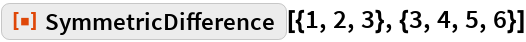Out=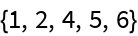Define three lists:

 In:=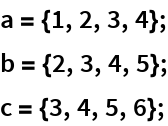Here are their Union and Intersection:

 In:=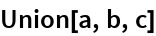Out=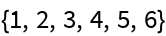In:=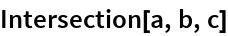Out=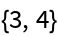Here is their symmetric difference:

 In:=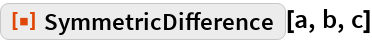Out=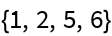### Possible Issues

First define four regions:

 In:=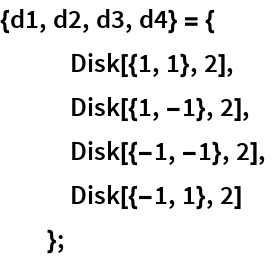The function RegionSymmetricDifference is like MultisetSymmetricDifference, not SymmetricDifference:

 In:=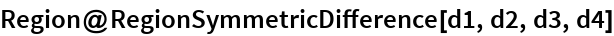Out=This construction is like SymmetricDifference:

 In:=Out=